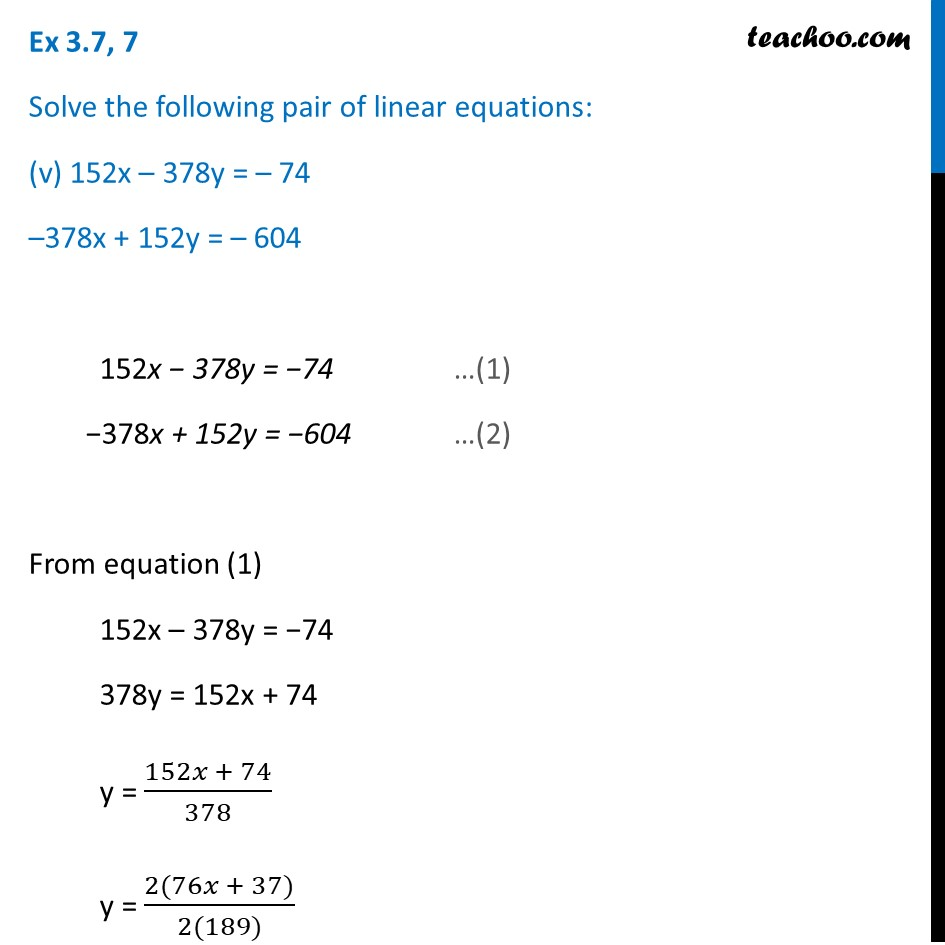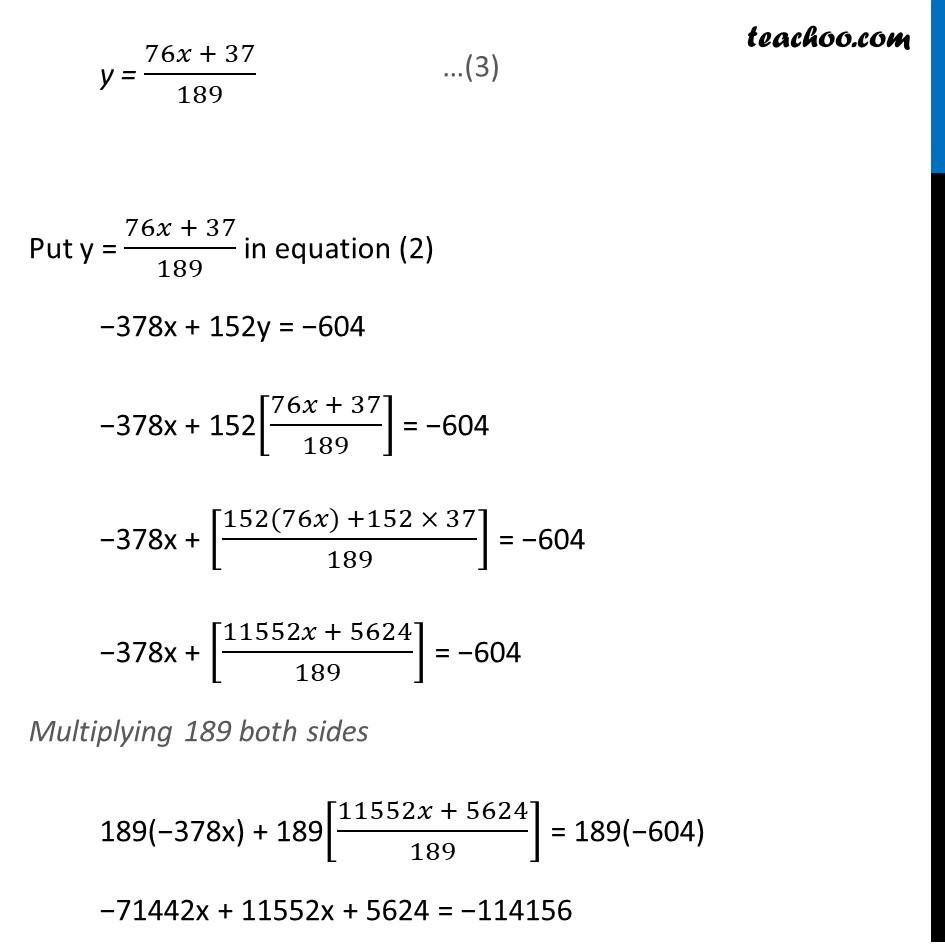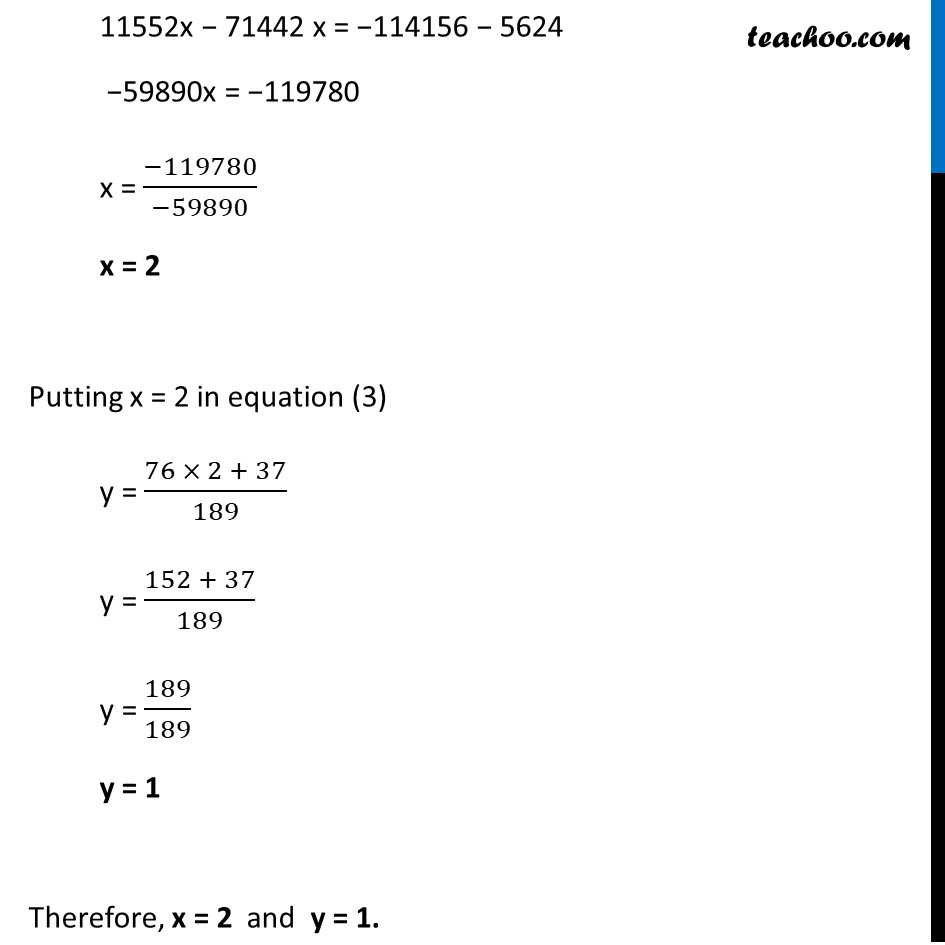1. Chapter 3 Class 10 Pair of Linear Equations in Two Variables (Term 1)
2. Serial order wise
3. Ex.3.7 (Optional)

Transcript

Ex 3.7, 7 Solve the following pair of linear equations: (v) 152x – 378y = – 74 –378x + 152y = – 604 152x − 378y = −74 …(1) −378x + 152y = −604 …(2) From equation (1) 152x – 378y = −74 378y = 152x + 74 y = (152𝑥 + 74)/378 y = (2(76𝑥 + 37))/(2(189)) y = (76𝑥 + 37)/189 Put y = (76𝑥 + 37)/189 in equation (2) −378x + 152y = −604 −378x + 152[(76𝑥 + 37)/189] = −604 −378x + [(152(76𝑥) +152 × 37)/189] = −604 −378x + [(11552𝑥 + 5624)/189] = −604 Multiplying 189 both sides 189(−378x) + 189[(11552𝑥 + 5624)/189] = 189(−604) −71442x + 11552x + 5624 = −114156 11552x − 71442 x = −114156 − 5624 −59890x = −119780 x = (−119780)/(−59890) x = 2 Putting x = 2 in equation (3) y = (76 × 2 + 37)/189 y = (152 + 37)/189 y = 189/189 y = 1 Therefore, x = 2 and y = 1.